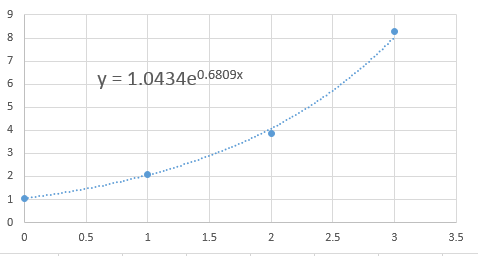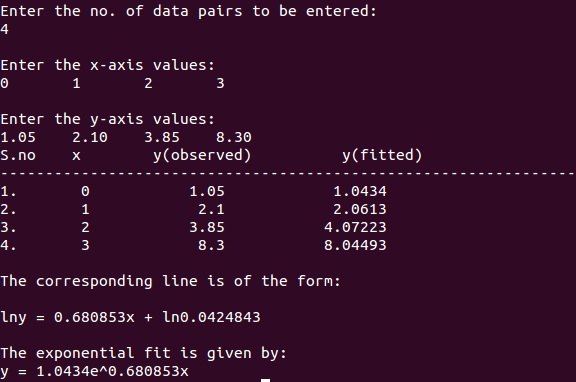# C++ Program for Exponential Fitting (Least Squares)

For a better written but C version of the program go here: https://www.bragitoff.com/2018/06/exponential-fitting-c-program/

```  //Exponential Fit
#include<iostream>
#include<iomanip>
#include<cmath>
using namespace std;
int main()
{
int i,j,k,n;
cout<<"\nEnter the no. of data pairs to be entered:\n";        //To find the size of arrays
cin>>n;
double x[n],y[n],lny[n],a,b,c;
cout<<"\nEnter the x-axis values:\n";                //Input x-values(observed)
for (i=0;i<n;i++)
cin>>x[i];
cout<<"\nEnter the y-axis values:\n";                //Input y-values(observed)
for (i=0;i<n;i++)
cin>>y[i];
for (i=0;i<n;i++)                        //Calculate the values of ln(yi)
lny[i]=log(y[i]);
double xsum=0,x2sum=0,ysum=0,xysum=0;                //variables for sums/sigma of xi,yi,xi^2,xiyi etc
for (i=0;i<n;i++)
{
xsum=xsum+x[i];                        //calculate sigma(xi)
ysum=ysum+lny[i];                        //calculate sigma(yi)
x2sum=x2sum+pow(x[i],2);                //calculate sigma(x^2i)
xysum=xysum+x[i]*lny[i];                    //calculate sigma(xi*yi)
}
a=(n*xysum-xsum*ysum)/(n*x2sum-xsum*xsum);            //calculate slope(or the the power of exp)
b=(x2sum*ysum-xsum*xysum)/(x2sum*n-xsum*xsum);            //calculate intercept
c=pow(2.71828,b);                        //since b=ln(c)
double y_fit[n];                        //an array to store the new fitted values of y
for (i=0;i<n;i++)
y_fit[i]=c*pow(2.71828,a*x[i]);                    //to calculate y(fitted) at given x points
cout<<"S.no"<<setw(5)<<"x"<<setw(19)<<"y(observed)"<<setw(19)<<"y(fitted)"<<endl;
cout<<"-----------------------------------------------------------------\n";
for (i=0;i<n;i++)
cout<<i+1<<"."<<setw(8)<<x[i]<<setw(15)<<y[i]<<setw(18)<<y_fit[i]<<endl;//print a table of x,y(obs.) and y(fit.)
cout<<"\nThe corresponding line is of the form:\n\nlny = "<<a<<"x + ln"<<b<<endl;
cout<<"\nThe exponential fit is given by:\ny = "<<c<<"e^"<<a<<"x\n";
return 0;
}
```[wpedon id="7041" align="center"]

## 9 thoughts on “C++ Program for Exponential Fitting (Least Squares)”

1. Dear Manas Sharma,
thanks a lot for the code reported above, was really useful for me.
I’m writing you because I would ask if you have some code for the double exponential fitting.
Just to be clear, a code that fit the series of data [x,y] with the follow model:
y(x)=a*exp(b*x) + c*exp(d*x)
To perform that fitting in Matlab is realy easy using the function “fit(X,Y,’exp2′)”, but is really difficult to find that code in C++

I will appreciate very much your help
Thanks a lot
Marco

1. Hi Marco,
Well, I haven’t tried it yet.
But sure I could do some research and write a code for that.
I am pretty busy at the moment, so it may take me a 2-3 days for that.

Feel free to ask anything you want to know.
I am happy to help.

Thanks!

1. Hi Manas,
thanks a lot for your support!
Best Regards
Marco

2. Hi Manas,

Thanks for the clear explanation on Exponential Fitting code.

Do you have any 2nd order exponential fitting program?

This is very useful to me

Thanks!

1. Hi there,
Thanks for your comment.
I am not really sure what you mean by 2nd order exponential fitting program.
Could you please explain it to me.
Also you can maybe check out some books on Numerical Methods to learn how to go about finding the equation for the fit using the Least Square Approximation.

3. Hi Manas,

2nd order exponential fitting is similar to Marco’s question

“ust to be clear, a code that fit the series of data [x,y] with the follow model:
y(x)=a*exp(b*x) + c*exp(d*x)”

Thank you for your help

Best Regards

4. Why don’t I see the entire code? Can you please update it 🙂

5. Hi Manas,

your fit was very useful for me.
Do you have a version with a constant in the exponential fit, like y = a * exp(b*x) + c?

Best Regards